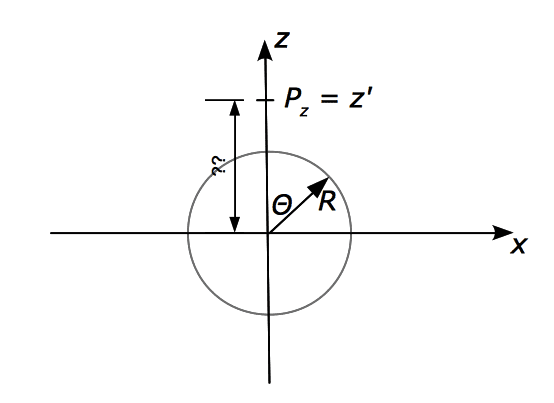# Calculate dipole moment

mzh
In the below figure, I'm supposed to express the z-coordinate of the point $P$, $z'$, by the angle $\theta$Does this work out as $z' = R\cdot \cos \theta$? If so, I can't see why...

Please give me a hint on this.

Muphrid

It pretty clearly says $P_z = z'$. Where is P?

mzh

It pretty clearly says $P_z = z'$. Where is P?

Sorry, $P$ "is" at [¡tex]P_z[/itex]. Its the z-component of the point.

Muphrid

If this is exactly the information you were given, then I see no way to solve the problem. $z'$ could be $R + 1$ or $R+1000$ and it wouldn't affect anything. Is this diagram the only information you were given, or is it constructed to match some sort of word problem?

Edit: most often, the angle is supposed to tell you the angle between the z-axis and the vector point $P$. It's unusual to say $P_z = z'$ and then to say P lies on the z-axis.

mzh

If this is exactly the information you were given, then I see no way to solve the problem. $z'$ could be $R + 1$ or $R+1000$ and it wouldn't affect anything. Is this diagram the only information you were given, or is it constructed to match some sort of word problem?

Edit: most often, the angle is supposed to tell you the angle between the z-axis and the vector point $P$. It's unusual to say $P_z = z'$ and then to say P lies on the z-axis.

yes, I'm trying to picture the problem. $P$ lies on the z-axis because the charge distribution is symmetric around z, and I indicated its z-component as $P_z$ and set it arbitrarily to $z'$.

It comes from Griffiths, Electrodynamics prob. 3.28a, where one is supposed to calculate the dipole moment of a surface of radius $R$, carrying surface charge density $\sigma=k \cos \theta$, the ansatz should be that $\vec{p} = \int (R \cos \theta) (k \cos \theta) R^2 \sin \theta d\theta d\phi$ but I can't figure out how to get the factor $(R \cos \theta)$. How do I get this factor?

Muphrid

Usually one computes the dipole moment at the center of a neutral charge distribution. Are you being asked for the dipole field at the point P?

Staff Emeritus
Homework Helper

This thread seems to be more about physics than the math. So I'm moving it.

mzh

Usually one computes the dipole moment at the center of a neutral charge distribution. Are you being asked for the dipole field at the point P?

the dipole moment for that matter...
apparently, by symmetry (which i do can see), the dipole moment $p$ only has z-component:

$\vec{p} = p \hat{\vec{z}}$, $p=\int z \rho d\tau \rightarrow p = \int z \sigma da$.

Which, considering the surface charge density i wrote previously, can be written as
$p= \int (R \cos \theta) (k\cos \theta) R^2 \sin \theta d\phi d\theta$. Where does the factor $(R \cos \theta)$ come from? It must be $z$, but I can't see how it relates to $z$.

Muphrid
I said specifically dipole field instead of dipole moment because the dipole moment is the same everywhere when there's no net charge in the system. So you can find the dipole moment about the origin, and that's all you need. Hence, $R \cos \theta$ is just the z-component of the distance from the origin to a point on the surface. You use the origin as the reference point because it's easier and because you know the dipole moment should be the same everywhere.

Still, I think you would be best served typing out the whole question. I feel very uncertain about what you're actually being asked.

mzh
I said specifically dipole field instead of dipole moment because the dipole moment is the same everywhere when there's no net charge in the system. So you can find the dipole moment about the origin, and that's all you need. Hence, $R \cos \theta$ is just the z-component of the distance from the origin to a point on the surface. You use the origin as the reference point because it's easier and because you know the dipole moment should be the same everywhere.

Still, I think you would be best served typing out the whole question. I feel very uncertain about what you're actually being asked.

Thanks for your comment. I'm still in the process of appreciating the subtilities of these points...

the exact wording is as:
[For a spherical shell] of radius $R$, which carries a surface charge $\sigma = k \cos \theta$, calculate the dipole moment of this surface charge distribution.